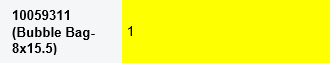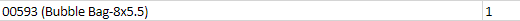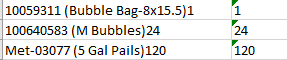# Code erasing numbers in datatable columns

Hi there, we have our code configured to grab an email body, perform string operations to separate into columns, and insert into a datatable. However, we are currently experiencing trouble with the code trimming numbers. I have attached examples below:

This first screenshot is from the email body:The second screenshot is from the datatable:In this case, if the quantity has a single digit number, it will erase all instances of that number in the first datatable column.

Any input as to a) what can be causing this and b) how we can fix this issue would be greatly appreciated.

Buddy if possible can i see the code a screenshot

Cheers

1 Like

We’re not 100% sure which code is causing this, but we believe it may be one of the two following codes that we have in our process:

{Regex.Replace(Line.Replace(Quantity,“”),“\t”,“”).Trim,Quantity}
or
{Regex.Replace(Line,“\t”,“”),Quantity}

In this case, Quantity is the second column in the datatable

This line here says to replace the quatity which is 1 in your example. So the first column will not have 1 in it anymore. So, I’m not sure why you are doing that. The second line of code you have there is correct and should only replace tabs.

does that help?

Yes, that does. This line is part of an else condition to where if String.IsNullOrEmpty(Quantity.Trim) ← if the row’s value in the second column contains any values, it adds a data row where {Regex.Replace(Line.Replace(Quantity,“”),“\t”,“”).Trim,Quantity} is in the ArrayRow Input. Would you have suggestions as to how to reconfigure it to prevent this?

From what you said,

if the row’s value in the second column contains any values, it adds a data row where {Regex.Replace(Line.Replace(Quantity,“”),“\t”,“”).Trim,Quantity}

Therefore, it will replace Quantity value in the first column with `Line.Replace(Quantity,"")`

So, to not do that, then take that part out, like:
`{Regex.Replace(Line,"\t",""),Quantity}`

1 Like

I’m sorry, I think that I worded my message incorrectly. I have the if statement set up to where:

If: if String.IsNullOrEmpty(Quantity.Trim)

Then: {Regex.Replace(Line,“\t”,“”),Quantity}
Else: {Regex.Replace(Line.Replace(Quantity,“”),“\t”,“”).Trim,Quantity}

However, if I use the {Regex.Replace(Line,“\t”,“”),Quantity} in the else statement like you suggested, the following appears:To where the values in the second column are duplicated in the first column.

I actually found the solution, I need to reconfigure the else statement to the following:
{Regex.Replace(Regex.Split(Line,“\t”)(0),“\t”,“”).Trim,Quantity}

1 Like

I see… so what you actually want to do is replace the last value that is the quantity.

You can use Regex for that.
Something like this:
`{Regex.Replace(Regex.Replace(Line.Trim,Quantity+"\$",""),"\t","").Trim,Quantity}`
the pattern used is Quantity+“\$”, which says the quantity that is at the very end of the string.

Regards.

1 Like

This topic was automatically closed 3 days after the last reply. New replies are no longer allowed.